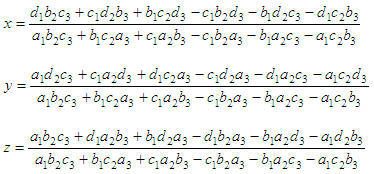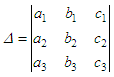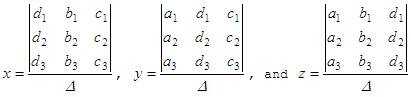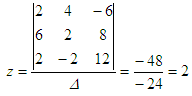# Cramer's Rule

The method of solution of linear equations by determinants is called Cramer's Rule. This rule for linear equations in 3 unknowns is a method of solving -by determinants- the following equations for x, y, z

a1x + b1y + c1z = d1
a2x + b2y + c2z = d2
a3x + b3y + c3z = d3

If we
analytically solve the equations above, we obtainIfis the determinant of coefficients of x, y, z and is

assumed not equal to zero, then we may re-write the values asThe solution involving determinants is easy to remember if you keep in mind these simple ideas:

• The denominators are given by the determinant in which the elements are the coefficients of x, y and z, arranged as in the original given equations.

• The numerator in the solution for any variable is the same as the determinant of the coefficients with the exception that the column of coefficients of the unknown to be determined is replaced by the column of constants on the right side of the original equations.

That is, for the first variable, you substitute the first column of the determinant with the constants on the right; for the second variable, you substitute the second column with the constants on the rigth, and so on...

Example:

Solve this system using Cramer’s Rule

2x + 4y – 2z = -6
6x + 2y + 2z = 8
2x – 2y + 4z = 12For x, take the determinant above and replace the first column by the constants on the right of the system. Then, divide this by the determinant:For y, replace the second column by the constants on the right of the system. Then, divide it by the determinant:For z, replace the third column by the constants on the right of the system. Then, divide it by the determinant:You just solved ths system!

In Matlab, it’s even easier. You can solve the system with just one instruction.

Let D be the matrix of just the coefficients of the variables:

D = [2  4 -2;
6  2  2;
2 -2  4];

Let b be the column vector of the constants on the rigth of the system :

b = [-6 8 12]'; % the apostrophe is used to transpose a vector

Find the column vector of the unknowns by 'left dividing' D by b (use the backslash), like this:

variables = D\b

And Matlab response is:

variables =
1.0000
-1.0000
2.0000

 Top Determinants